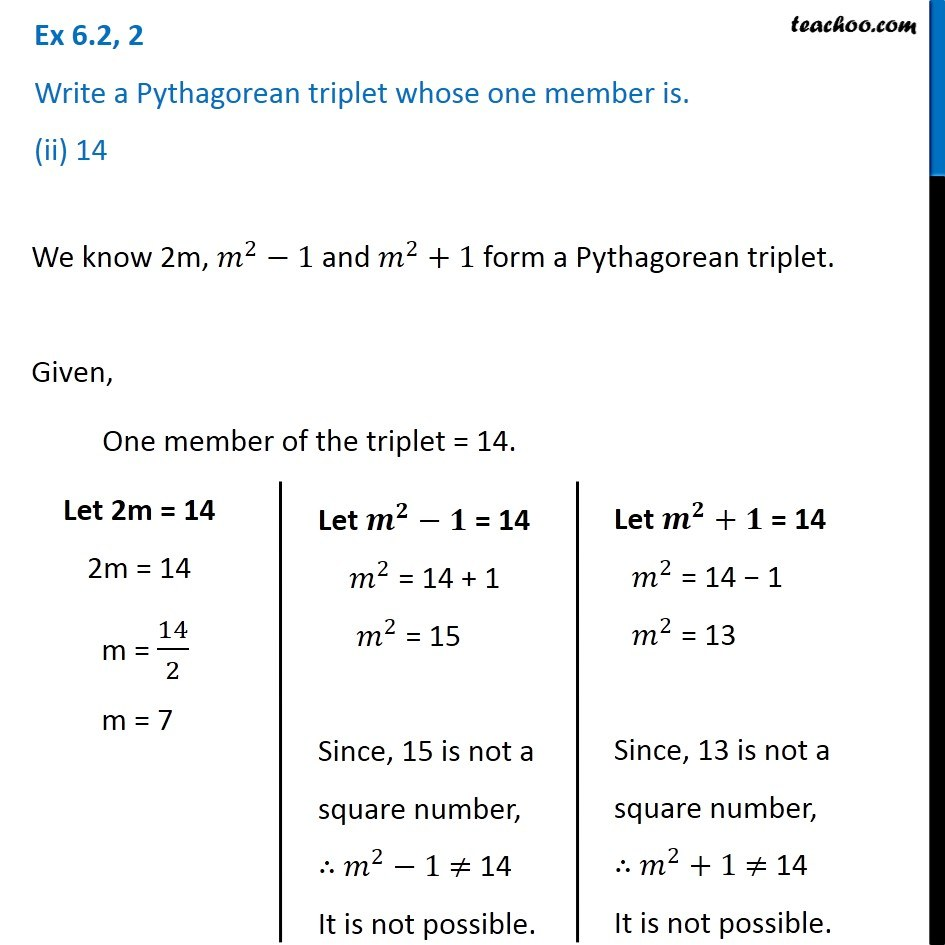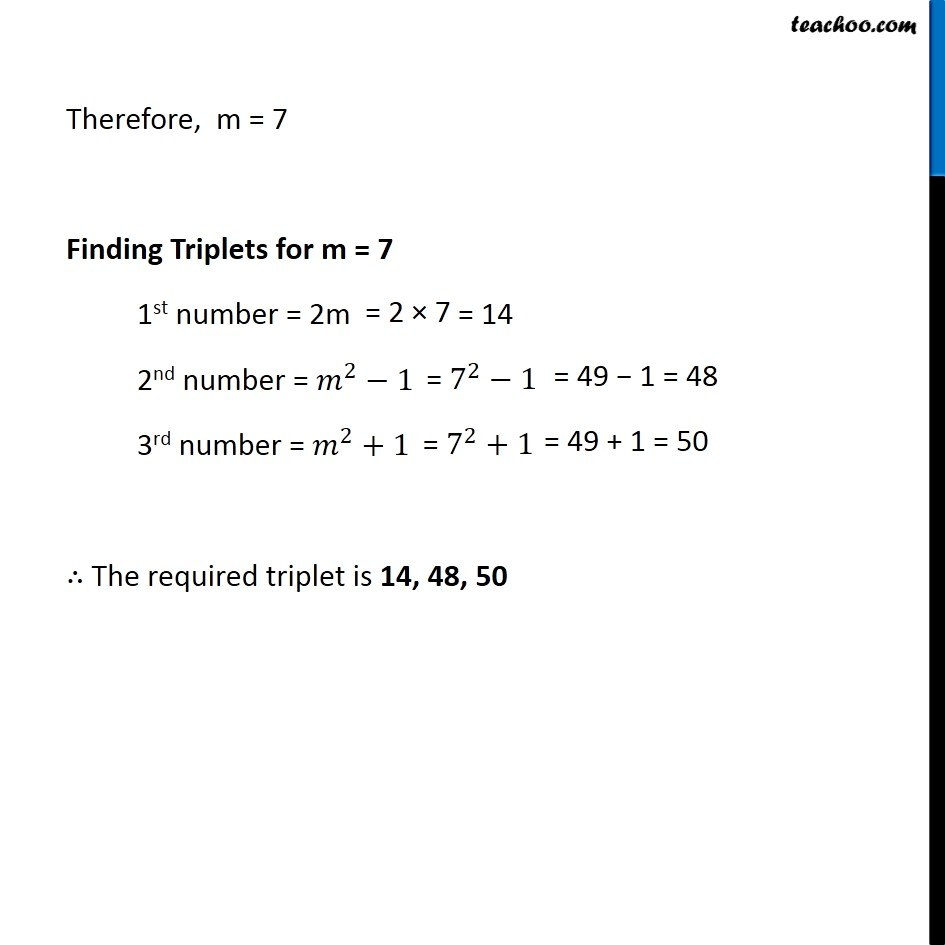Ex 6.2

Chapter 6 Class 8 Squares and Square Roots
Serial order wise549 people joined Teachoo Black. What are you waiting for?

### Transcript

Ex 6.2, 2 Write a Pythagorean triplet whose one member is. (ii) 14We know 2m, 𝑚^2−1 and 𝑚^2+1 form a Pythagorean triplet. Given, One member of the triplet = 14. Let 2m = 14 2m = 14 m = 14/2 m = 7 Let 𝒎^𝟐−𝟏" = 14" 𝑚^2 = 14 + 1 𝑚^2 = 15 Since, 15 is not a square number, ∴ 𝑚^2−1 ≠ 14 It is not possible. Let 𝒎^𝟐+𝟏 = 14 𝑚^2 = 14 − 1 𝑚^2 = 13 Since, 13 is not a square number, ∴ 𝑚^2+1 ≠ 14 It is not possible. Therefore, m = 7 Finding Triplets for m = 7 1st number = 2m 2nd number = 𝑚^2−1 3rd number = 𝑚^2+1 ∴ The required triplet is 14, 48, 50